# INTRODUCTION TO C++.pptx

1 de Jun de 2023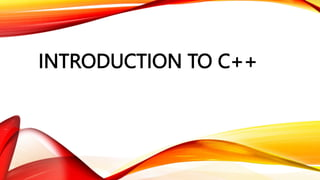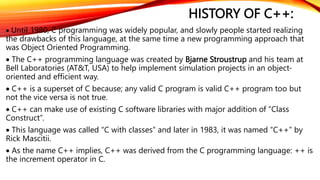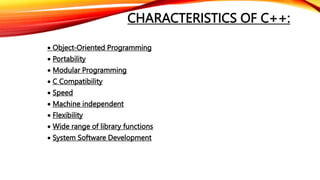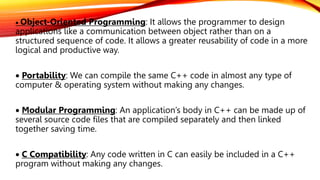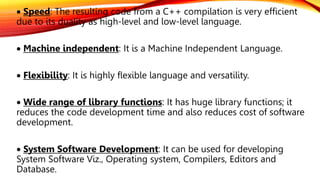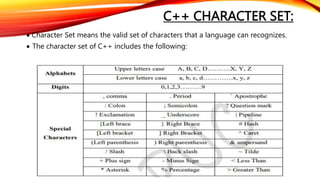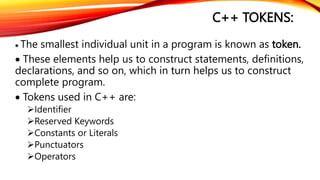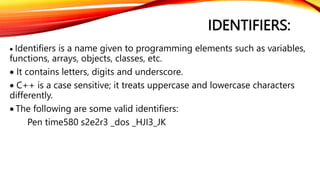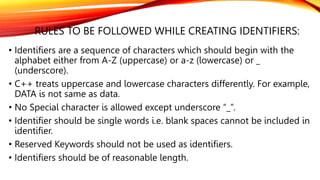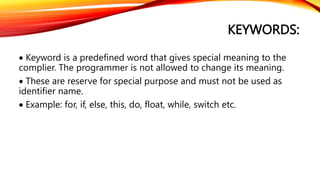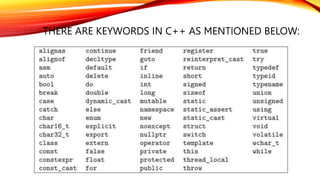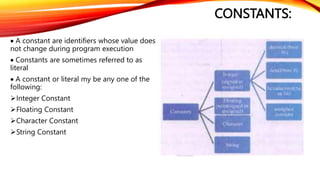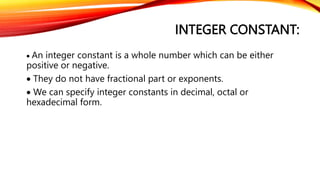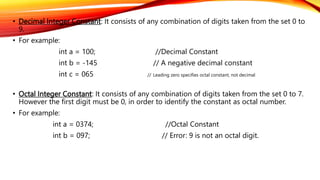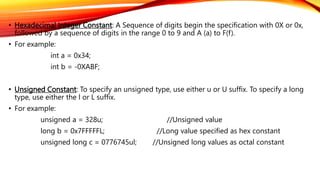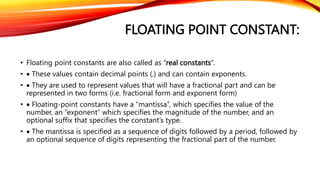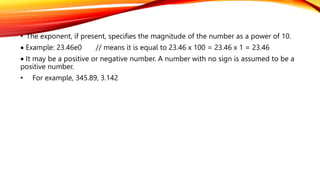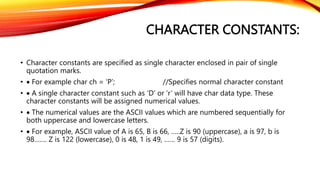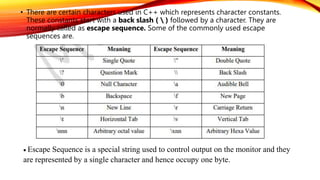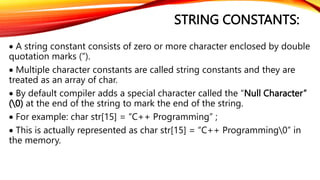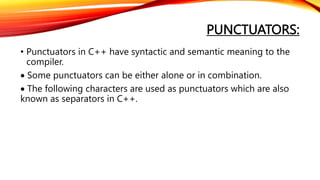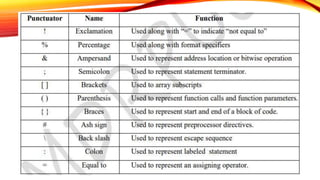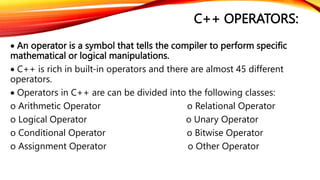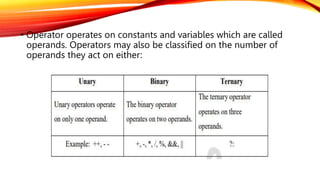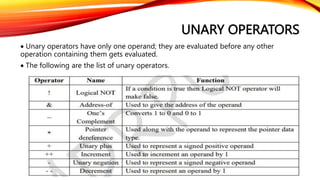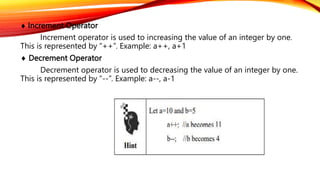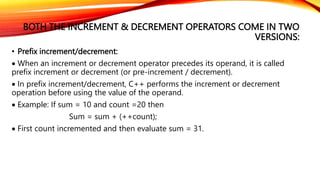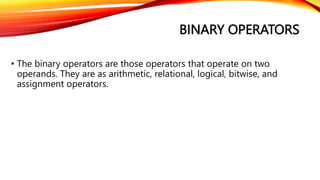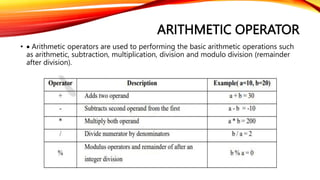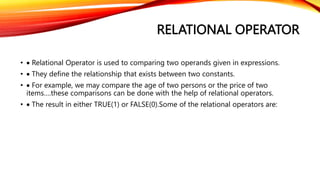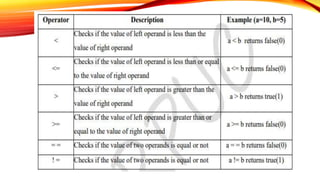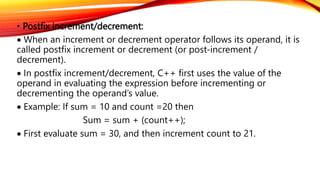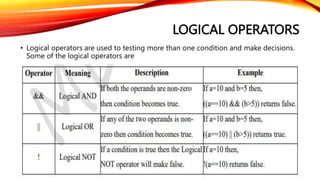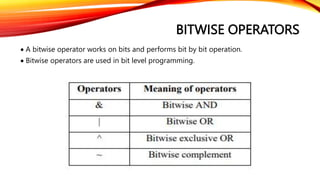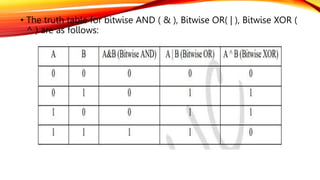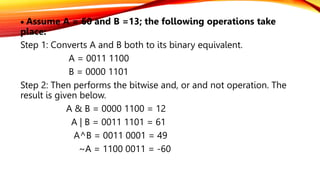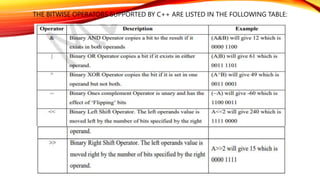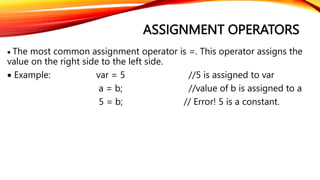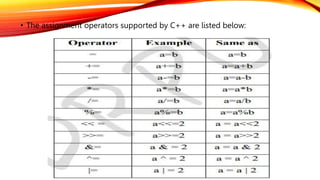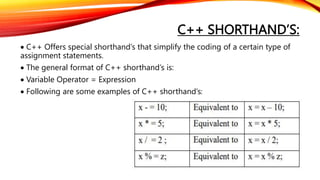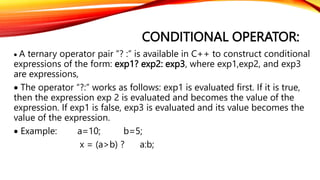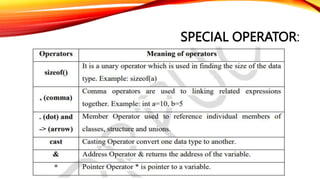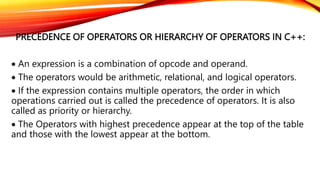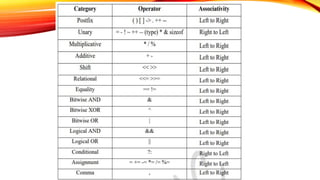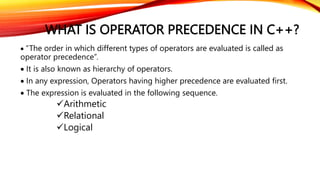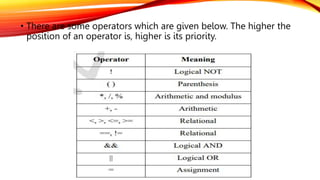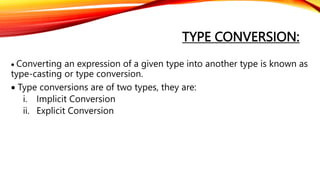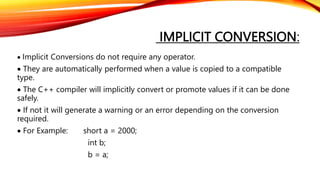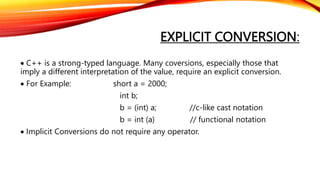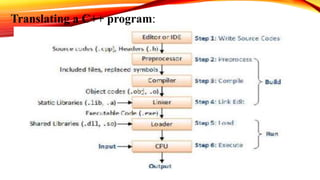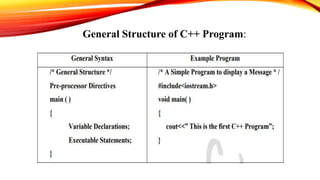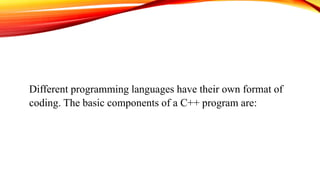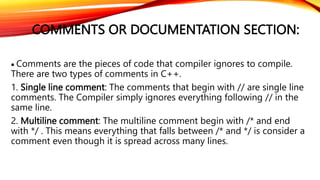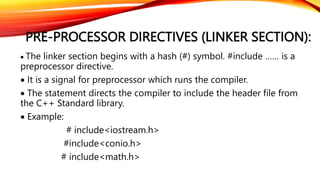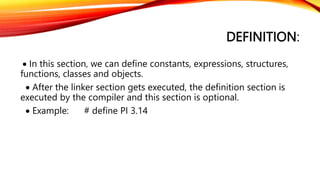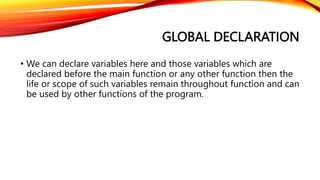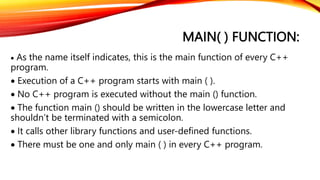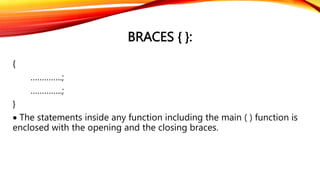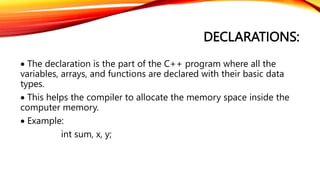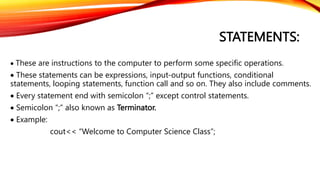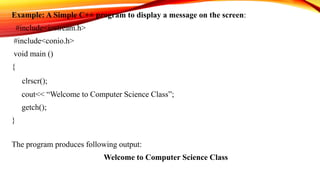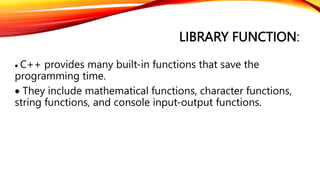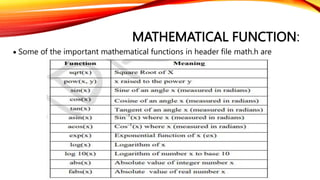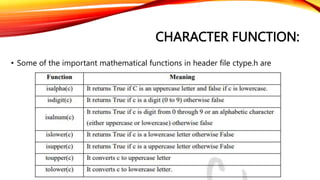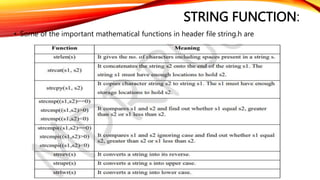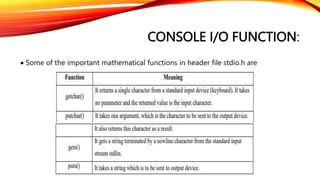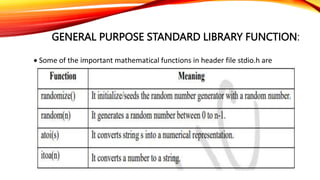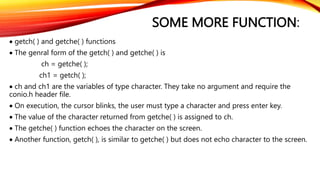1 de 68

### INTRODUCTION TO C++.pptx

• 1. INTRODUCTION TO C++
• 2. HISTORY OF C++:  Until 1980, C programming was widely popular, and slowly people started realizing the drawbacks of this language, at the same time a new programming approach that was Object Oriented Programming.  The C++ programming language was created by Bjarne Stroustrup and his team at Bell Laboratories (AT&T, USA) to help implement simulation projects in an object- oriented and efficient way.  C++ is a superset of C because; any valid C program is valid C++ program too but not the vice versa is not true.  C++ can make use of existing C software libraries with major addition of “Class Construct”.  This language was called “C with classes” and later in 1983, it was named “C++” by Rick Mascitii.  As the name C++ implies, C++ was derived from the C programming language: ++ is the increment operator in C.
• 3. CHARACTERISTICS OF C++:  Object-Oriented Programming  Portability  Modular Programming  C Compatibility  Speed  Machine independent  Flexibility  Wide range of library functions  System Software Development
• 4.  Object-Oriented Programming: It allows the programmer to design applications like a communication between object rather than on a structured sequence of code. It allows a greater reusability of code in a more logical and productive way.  Portability: We can compile the same C++ code in almost any type of computer & operating system without making any changes.  Modular Programming: An application’s body in C++ can be made up of several source code files that are compiled separately and then linked together saving time.  C Compatibility: Any code written in C can easily be included in a C++ program without making any changes.
• 5.  Speed: The resulting code from a C++ compilation is very efficient due to its duality as high-level and low-level language.  Machine independent: It is a Machine Independent Language.  Flexibility: It is highly flexible language and versatility.  Wide range of library functions: It has huge library functions; it reduces the code development time and also reduces cost of software development.  System Software Development: It can be used for developing System Software Viz., Operating system, Compilers, Editors and Database.
• 6. C++ CHARACTER SET:  Character Set means the valid set of characters that a language can recognizes.  The character set of C++ includes the following:
• 7. C++ TOKENS:  The smallest individual unit in a program is known as token.  These elements help us to construct statements, definitions, declarations, and so on, which in turn helps us to construct complete program.  Tokens used in C++ are: Identifier Reserved Keywords Constants or Literals Punctuators Operators
• 8. IDENTIFIERS:  Identifiers is a name given to programming elements such as variables, functions, arrays, objects, classes, etc.  It contains letters, digits and underscore.  C++ is a case sensitive; it treats uppercase and lowercase characters differently.  The following are some valid identifiers: Pen time580 s2e2r3 _dos _HJI3_JK
• 9. RULES TO BE FOLLOWED WHILE CREATING IDENTIFIERS: • Identifiers are a sequence of characters which should begin with the alphabet either from A-Z (uppercase) or a-z (lowercase) or _ (underscore). • C++ treats uppercase and lowercase characters differently. For example, DATA is not same as data. • No Special character is allowed except underscore “_”. • Identifier should be single words i.e. blank spaces cannot be included in identifier. • Reserved Keywords should not be used as identifiers. • Identifiers should be of reasonable length.
• 10. KEYWORDS:  Keyword is a predefined word that gives special meaning to the complier. The programmer is not allowed to change its meaning.  These are reserve for special purpose and must not be used as identifier name.  Example: for, if, else, this, do, float, while, switch etc.
• 11. THERE ARE KEYWORDS IN C++ AS MENTIONED BELOW:
• 12. CONSTANTS:  A constant are identifiers whose value does not change during program execution  Constants are sometimes referred to as literal  A constant or literal my be any one of the following: Integer Constant Floating Constant Character Constant String Constant
• 13. INTEGER CONSTANT:  An integer constant is a whole number which can be either positive or negative.  They do not have fractional part or exponents.  We can specify integer constants in decimal, octal or hexadecimal form.
• 14. • Decimal Integer Constant: It consists of any combination of digits taken from the set 0 to 9. • For example: int a = 100; //Decimal Constant int b = -145 // A negative decimal constant int c = 065 // Leading zero specifies octal constant, not decimal • Octal Integer Constant: It consists of any combination of digits taken from the set 0 to 7. However the first digit must be 0, in order to identify the constant as octal number. • For example: int a = 0374; //Octal Constant int b = 097; // Error: 9 is not an octal digit.
• 15. • Hexadecimal Integer Constant: A Sequence of digits begin the specification with 0X or 0x, followed by a sequence of digits in the range 0 to 9 and A (a) to F(f). • For example: int a = 0x34; int b = -0XABF; • Unsigned Constant: To specify an unsigned type, use either u or U suffix. To specify a long type, use either the l or L suffix. • For example: unsigned a = 328u; //Unsigned value long b = 0x7FFFFFL; //Long value specified as hex constant unsigned long c = 0776745ul; //Unsigned long values as octal constant
• 16. FLOATING POINT CONSTANT: • Floating point constants are also called as “real constants”. •  These values contain decimal points (.) and can contain exponents. •  They are used to represent values that will have a fractional part and can be represented in two forms (i.e. fractional form and exponent form) •  Floating-point constants have a “mantissa”, which specifies the value of the number, an “exponent” which specifies the magnitude of the number, and an optional suffix that specifies the constant’s type. •  The mantissa is specified as a sequence of digits followed by a period, followed by an optional sequence of digits representing the fractional part of the number.
• 17. • The exponent, if present, specifies the magnitude of the number as a power of 10.  Example: 23.46e0 // means it is equal to 23.46 x 100 = 23.46 x 1 = 23.46  It may be a positive or negative number. A number with no sign is assumed to be a positive number. • For example, 345.89, 3.142
• 18. CHARACTER CONSTANTS: • Character constants are specified as single character enclosed in pair of single quotation marks. •  For example char ch = ‘P’; //Specifies normal character constant •  A single character constant such as ‘D’ or ‘r’ will have char data type. These character constants will be assigned numerical values. •  The numerical values are the ASCII values which are numbered sequentially for both uppercase and lowercase letters. •  For example, ASCII value of A is 65, B is 66, …..Z is 90 (uppercase), a is 97, b is 98……. Z is 122 (lowercase), 0 is 48, 1 is 49, …… 9 is 57 (digits).
• 19. • There are certain characters used in C++ which represents character constants. These constants start with a back slash ( ) followed by a character. They are normally called as escape sequence. Some of the commonly used escape sequences are.  Escape Sequence is a special string used to control output on the monitor and they are represented by a single character and hence occupy one byte.
• 20. STRING CONSTANTS:  A string constant consists of zero or more character enclosed by double quotation marks (“).  Multiple character constants are called string constants and they are treated as an array of char.  By default compiler adds a special character called the “Null Character” (0) at the end of the string to mark the end of the string.  For example: char str = “C++ Programming” ;  This is actually represented as char str = “C++ Programming0” in the memory.
• 21. PUNCTUATORS: • Punctuators in C++ have syntactic and semantic meaning to the compiler.  Some punctuators can be either alone or in combination.  The following characters are used as punctuators which are also known as separators in C++.
• 23. C++ OPERATORS:  An operator is a symbol that tells the compiler to perform specific mathematical or logical manipulations.  C++ is rich in built-in operators and there are almost 45 different operators.  Operators in C++ are can be divided into the following classes: o Arithmetic Operator o Relational Operator o Logical Operator o Unary Operator o Conditional Operator o Bitwise Operator o Assignment Operator o Other Operator
• 24. • Operator operates on constants and variables which are called operands. Operators may also be classified on the number of operands they act on either:
• 25. UNARY OPERATORS  Unary operators have only one operand; they are evaluated before any other operation containing them gets evaluated.  The following are the list of unary operators.
• 26.  Increment Operator Increment operator is used to increasing the value of an integer by one. This is represented by “++”. Example: a++, a+1  Decrement Operator Decrement operator is used to decreasing the value of an integer by one. This is represented by “--”. Example: a--, a-1
• 27. BOTH THE INCREMENT & DECREMENT OPERATORS COME IN TWO VERSIONS: • Prefix increment/decrement:  When an increment or decrement operator precedes its operand, it is called prefix increment or decrement (or pre-increment / decrement).  In prefix increment/decrement, C++ performs the increment or decrement operation before using the value of the operand.  Example: If sum = 10 and count =20 then Sum = sum + (++count);  First count incremented and then evaluate sum = 31.
• 28. BINARY OPERATORS • The binary operators are those operators that operate on two operands. They are as arithmetic, relational, logical, bitwise, and assignment operators.
• 29. ARITHMETIC OPERATOR •  Arithmetic operators are used to performing the basic arithmetic operations such as arithmetic, subtraction, multiplication, division and modulo division (remainder after division).
• 30. RELATIONAL OPERATOR •  Relational Operator is used to comparing two operands given in expressions. •  They define the relationship that exists between two constants. •  For example, we may compare the age of two persons or the price of two items….these comparisons can be done with the help of relational operators. •  The result in either TRUE(1) or FALSE(0).Some of the relational operators are:
• 32. • Postfix increment/decrement:  When an increment or decrement operator follows its operand, it is called postfix increment or decrement (or post-increment / decrement).  In postfix increment/decrement, C++ first uses the value of the operand in evaluating the expression before incrementing or decrementing the operand’s value.  Example: If sum = 10 and count =20 then Sum = sum + (count++);  First evaluate sum = 30, and then increment count to 21.
• 33. LOGICAL OPERATORS • Logical operators are used to testing more than one condition and make decisions. Some of the logical operators are
• 34. BITWISE OPERATORS  A bitwise operator works on bits and performs bit by bit operation.  Bitwise operators are used in bit level programming.
• 35. • The truth table for bitwise AND ( & ), Bitwise OR( | ), Bitwise XOR ( ^ ) are as follows:
• 36.  Assume A = 60 and B =13; the following operations take place: Step 1: Converts A and B both to its binary equivalent. A = 0011 1100 B = 0000 1101 Step 2: Then performs the bitwise and, or and not operation. The result is given below. A & B = 0000 1100 = 12 A | B = 0011 1101 = 61 A^B = 0011 0001 = 49 ~A = 1100 0011 = -60
• 37. THE BITWISE OPERATORS SUPPORTED BY C++ ARE LISTED IN THE FOLLOWING TABLE:
• 38. ASSIGNMENT OPERATORS  The most common assignment operator is =. This operator assigns the value on the right side to the left side.  Example: var = 5 //5 is assigned to var a = b; //value of b is assigned to a 5 = b; // Error! 5 is a constant.
• 39. • The assignment operators supported by C++ are listed below:
• 40. C++ SHORTHAND’S:  C++ Offers special shorthand’s that simplify the coding of a certain type of assignment statements.  The general format of C++ shorthand’s is:  Variable Operator = Expression  Following are some examples of C++ shorthand’s:
• 41. CONDITIONAL OPERATOR:  A ternary operator pair ”? :” is available in C++ to construct conditional expressions of the form: exp1? exp2: exp3, where exp1,exp2, and exp3 are expressions,  The operator “?:” works as follows: exp1 is evaluated first. If it is true, then the expression exp 2 is evaluated and becomes the value of the expression. If exp1 is false, exp3 is evaluated and its value becomes the value of the expression.  Example: a=10; b=5; x = (a>b) ? a:b;
• 42. SPECIAL OPERATOR:
• 43. PRECEDENCE OF OPERATORS OR HIERARCHY OF OPERATORS IN C++:  An expression is a combination of opcode and operand.  The operators would be arithmetic, relational, and logical operators.  If the expression contains multiple operators, the order in which operations carried out is called the precedence of operators. It is also called as priority or hierarchy.  The Operators with highest precedence appear at the top of the table and those with the lowest appear at the bottom.
• 45. WHAT IS OPERATOR PRECEDENCE IN C++?  “The order in which different types of operators are evaluated is called as operator precedence”.  It is also known as hierarchy of operators.  In any expression, Operators having higher precedence are evaluated first.  The expression is evaluated in the following sequence. Arithmetic Relational Logical
• 46. • There are some operators which are given below. The higher the position of an operator is, higher is its priority.
• 47. TYPE CONVERSION:  Converting an expression of a given type into another type is known as type-casting or type conversion.  Type conversions are of two types, they are: i. Implicit Conversion ii. Explicit Conversion
• 48. IMPLICIT CONVERSION:  Implicit Conversions do not require any operator.  They are automatically performed when a value is copied to a compatible type.  The C++ compiler will implicitly convert or promote values if it can be done safely.  If not it will generate a warning or an error depending on the conversion required.  For Example: short a = 2000; int b; b = a;
• 49. EXPLICIT CONVERSION:  C++ is a strong-typed language. Many coversions, especially those that imply a different interpretation of the value, require an explicit conversion.  For Example: short a = 2000; int b; b = (int) a; //c-like cast notation b = int (a) // functional notation  Implicit Conversions do not require any operator.
• 50. Translating a C++ program:
• 51. General Structure of C++ Program:
• 52. Different programming languages have their own format of coding. The basic components of a C++ program are:
• 53. COMMENTS OR DOCUMENTATION SECTION:  Comments are the pieces of code that compiler ignores to compile. There are two types of comments in C++. 1. Single line comment: The comments that begin with // are single line comments. The Compiler simply ignores everything following // in the same line. 2. Multiline comment: The multiline comment begin with /* and end with */ . This means everything that falls between /* and */ is consider a comment even though it is spread across many lines.
• 54. PRE-PROCESSOR DIRECTIVES (LINKER SECTION):  The linker section begins with a hash (#) symbol. #include …… is a preprocessor directive.  It is a signal for preprocessor which runs the compiler.  The statement directs the compiler to include the header file from the C++ Standard library.  Example: # include<iostream.h> #include<conio.h> # include<math.h>
• 55. DEFINITION:  In this section, we can define constants, expressions, structures, functions, classes and objects.  After the linker section gets executed, the definition section is executed by the compiler and this section is optional.  Example: # define PI 3.14
• 56. GLOBAL DECLARATION • We can declare variables here and those variables which are declared before the main function or any other function then the life or scope of such variables remain throughout function and can be used by other functions of the program.
• 57. MAIN( ) FUNCTION:  As the name itself indicates, this is the main function of every C++ program.  Execution of a C++ program starts with main ( ).  No C++ program is executed without the main () function.  The function main () should be written in the lowercase letter and shouldn’t be terminated with a semicolon.  It calls other library functions and user-defined functions.  There must be one and only main ( ) in every C++ program.
• 58. BRACES { }: { …………..; …………..; }  The statements inside any function including the main ( ) function is enclosed with the opening and the closing braces.
• 59. DECLARATIONS:  The declaration is the part of the C++ program where all the variables, arrays, and functions are declared with their basic data types.  This helps the compiler to allocate the memory space inside the computer memory.  Example: int sum, x, y;
• 60. STATEMENTS:  These are instructions to the computer to perform some specific operations.  These statements can be expressions, input-output functions, conditional statements, looping statements, function call and so on. They also include comments.  Every statement end with semicolon “;” except control statements.  Semicolon “;” also known as Terminator.  Example: cout<< “Welcome to Computer Science Class”;
• 61. Example: A Simple C++ program to display a message on the screen: #include<iostream.h> #include<conio.h> void main () { clrscr(); cout<< “Welcome to Computer Science Class”; getch(); } The program produces following output: Welcome to Computer Science Class
• 62. LIBRARY FUNCTION:  C++ provides many built-in functions that save the programming time.  They include mathematical functions, character functions, string functions, and console input-output functions.
• 63. MATHEMATICAL FUNCTION:  Some of the important mathematical functions in header file math.h are
• 64. CHARACTER FUNCTION: • Some of the important mathematical functions in header file ctype.h are
• 65. STRING FUNCTION: • Some of the important mathematical functions in header file string.h are
• 66. CONSOLE I/O FUNCTION:  Some of the important mathematical functions in header file stdio.h are
• 67. GENERAL PURPOSE STANDARD LIBRARY FUNCTION:  Some of the important mathematical functions in header file stdio.h are
• 68. SOME MORE FUNCTION:  getch( ) and getche( ) functions  The genral form of the getch( ) and getche( ) is ch = getche( ); ch1 = getch( );  ch and ch1 are the variables of type character. They take no argument and require the conio.h header file.  On execution, the cursor blinks, the user must type a character and press enter key.  The value of the character returned from getche( ) is assigned to ch.  The getche( ) function echoes the character on the screen.  Another function, getch( ), is similar to getche( ) but does not echo character to the screen.